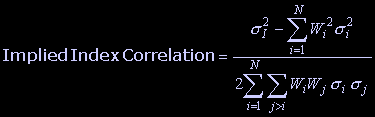## Thursday, September 29, 2011

### The Dispersion Trade (Volatility Stat Arb)The dispersion trade is about exploiting option volatilities, or implied correlation, between indices and their component stocks. It's similar to an index arbitrage between futures and spot, just that now we're looking at their options. Anyone looking into actual "Volatility Arbitrage", this is it. The wikipedia definition is way off, not an arb at all.

Here we can see why the implied correlation simply represents a normalized difference of Index vs. composite component variance values.EGAR Tech Tutorial offers a pretty detailed explanation of the trade. So to save myself from having to write all this out, here're some key points of the trade:

"

Volatility Dispersion Strategy is considered to be one of the best working strategies in sophisticated analytics. It can be explained by the fact, that historically index volatility has traded rich, while individual stock volatility has been fairly priced. Thus the dispersion strategy allows traders to profit from price differences using index options and offsetting options on individual stocks.

The dispersion strategy typically consists of short selling options on a stock index while simultaneously buying options on the component stocks, i.e. leaves short correlation and long dispersion. The reverse dispersion strategy consists of buying options on a stock index and selling options on the component stocks.

The success of the Volatility Dispersion Strategy lies in determining whether the time is right to do a dispersion trade at all, and selecting the best possible stocks for the offsetting dispersion basket.

THE BEST TIME FOR THE DISPERSION STRATEGY
When selecting the best time to engage in the dispersion strategy, you should to pay attention to the following parameters:

IV Index of index
If the relation of actual implied volatility of index to historical volatility is greater than 1 and relatively high, it is good time for selling index options, since it means selling expensive options on the stock index.
If the relation of actual implied volatility of index to historical volatility is less than 1 and relatively low, it is good time for buying index options, since it means buying relatively cheap options.

Implied Index Correlation
Implied Index Correlation should not be too far from the maximum registered value, since the dispersion strategy works better if the implied volatility of the index is highly correlated with the implied volatilities of its stock components.

WtdCompIV/IV Index – first volatility level coefficient
The low value corresponds to high Implied Index Correlation. So the lower the value of the first volatility level coefficient, the better the time to engage in a dispersion strategy.

CorrWtdCompIV/IV Index – second volatility level coefficient is a better measure for for longer term trade.
If the second volatility level coefficient is less than one and relatively low, it means that theoretical performance of the index is less then implied by market and a trader can gain profit selling index options. Otherwise, if the second coefficient is greater than one and relatively high, it is a better time for buying index options.

CorrWtdCompHV/HV - third volatility level coefficient is a better measure for shorter term trade.
If the third volatility level coefficient is less than one and relatively low, it means that theoretical performance of the index is less then implied by market and a trader can gain profit selling index options. Otherwise, if the third coefficient is greater than one and relatively high, it is a better time for buying index options.

"